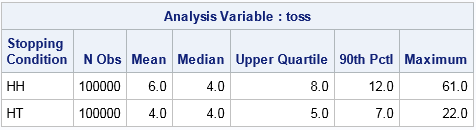I saw an interesting mathematical result in Wired magazine. The original article was about mathematical research into prime numbers, but the article included the following tantalizing fact:

If Alice tosses a [fair] coin until she sees a head followed by a tail, and Bob tosses a coin until he sees two heads in a row, then on average, Alice will require four tosses while Bob will require six tosses (try this at home!), even though head-tail and head-head have an equal chance of appearing after two coin tosses.

Yes, I would classify that result as counter-intuitive! When I read this paragraph, I knew I had to simulate these coin tosses in SAS.

### Why does head-tail happen sooner (on average)?

Define the "game" for Alice as a sequence of coin tosses that terminates in a head followed by a tail. For Bob, the game is a sequence of coin tosses that terminates in two consecutive heads.

You can see evidence that the result is true by looking at the possible sequence of tosses that end the game for Alice versus Bob. First consider the sequences for Alice that terminate with head-tail (HT). The following six sequences terminate in four or fewer tosses:

``` HT THT HHT THHT TTHT HHHT```

In contrast, among Bob's sequences that terminate with head-head (HH), only four require four or fewer tosses:

``` HH THH TTHH HTHH```

For Bob to "win" (that is, observe the sequence HH), he must first toss a head. On the next toss, if he obtains a head again, he wins. But if he observes a tail, he is must start over: He has to toss until a head appears, and the process continues.

In contrast, for Alice to "win" (that is, observe the sequence HT), she must first toss a head. If the next toss shows a tail, she wins. But if is a head, she doesn't start over. In fact, she is already halfway to winning on the subsequent toss.

This result is counterintuitive because a sequence is just as likely to terminate with HH as with HT. So Alice and Bob "win" equally often if they are observing flips of a single fair coin. However, the sequences for which Alice wins tend to be shorter than those for which Bob wins.

Click To Tweet

### A simulation of coin tosses

The following SAS DATA step simulates Alice and Bob tossing coins until they have each won 100,000 games. For each player, the program counts how many tosses are needed before the "winning" sequence occurs. A random Bernoulli number (0 or 1 with probability 0.5) simulates the coin toss.

```data CoinFlip(keep= player toss); call streaminit(123456789); label Player = "Stopping Condition"; do i = 1 to 100000; do player = "HT", "HH"; /* first Alice tosses; then Bob */ first = rand("Bernoulli", 0.5); /* 0 is Tail; 1 is Head */ toss = 1; done = 0; do until (done); second = rand("Bernoulli", 0.5); toss = toss + 1; /* how many tosses until done? */ if player = "HT" then /* if Alice, ... */ done = (first=1 & second=0); /* stop when HT appears */ else /* if Bob, ... */ done = (first=1 & second=1); /* stop when HH appears */ first = second; /* remember for next toss */ end; output; end; end; run;   proc means data=CoinFlip MAXDEC=1 mean median Q3 P90 max; class player; var toss; run;```The MEANS procedure displays statistics about the length of each game. The mean (expected) length of the game for Bob (HH) is 6 tosses. 75% of games were resolved in eight tosses or less and 90% required 12 or less. In the 100,000 simulated games, the longest game that Bob played required 61 tosses before it terminated. (The maximum game length varies with the random number seed.)

In contrast, Alice resolved her 100,000 games by using about 50% fewer tosses. The mean (expected) length of the game for Alice (HT) is 4 tosses. 75% of the games required five or fewer tosses and 90% were completed in seven or fewer tosses. In the 100,000 simulated games, the longest game that Alice played required 22 tosses.You can create a comparative histogram to compare the distribution of the game lengths. The distribution of the length of Bob's games is shown in the upper histogram. It has a long tail.

In contrast, the distribution of Alice's game lengths is shown in the bottom of the panel. The distribution drops off much more quickly than for Bob's games.

It is also enlightening to see the empirical cumulative distribution functions (ECDFs) for the number of tosses for each player. You can download a SAS program that creates the comparative histogram and the ECDFs. For skeptics, the program also contains a simulation that demonstrates that a sequence terminates with HH as often as it terminates with HT.

The result is not deep, but it reminds us that the human intuition gets confused by conditional probability. Like the classic the Monty Hall problem, simulation can convince us that a result is true, even when our intuition refuses to believe it.

The post Head-tail versus head-head: A counterintuitive property of coin tosses appeared first on The DO Loop.